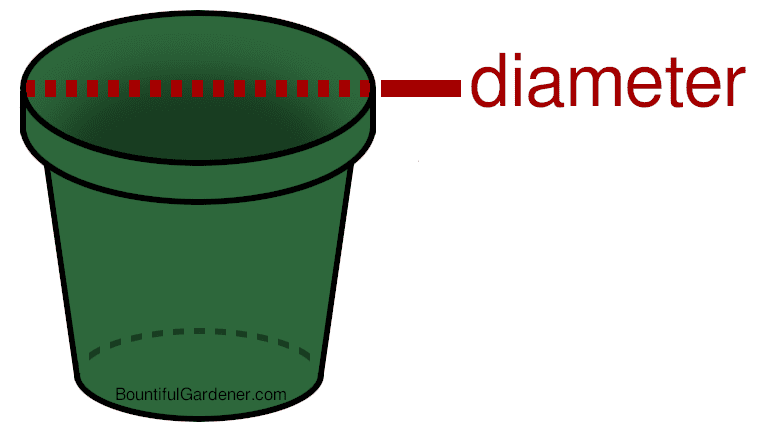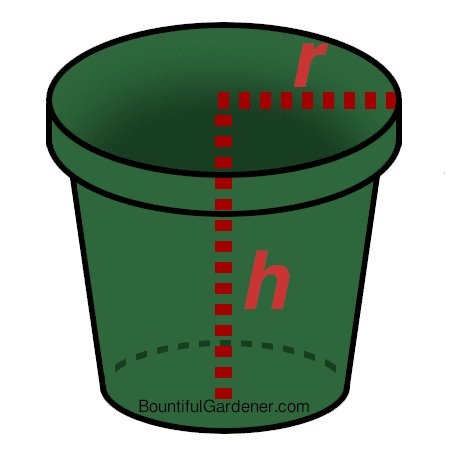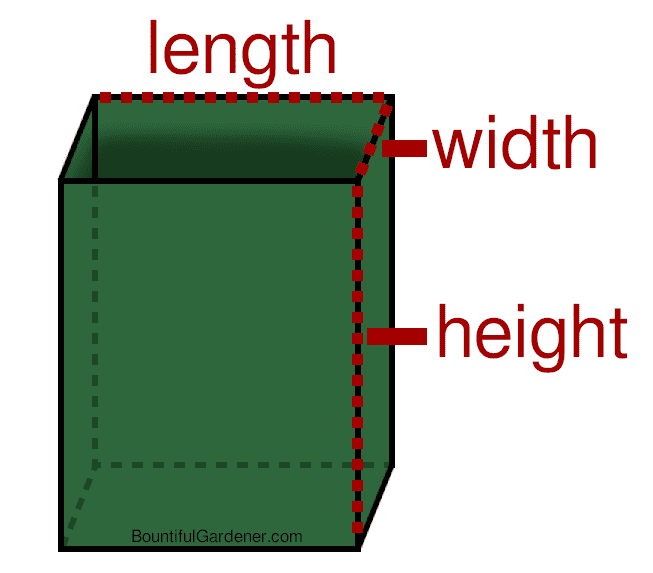# How to Measure Plant Pot Size (with Calculations)

Using the right pot size can mean the difference between having thriving plants with lots of room to spread their roots and struggling, root-bound ones that can’t reach their full potential. Some pots will already have the size written or stamped on them, usually on the side or bottom (especially nursery pots). So, why would you want to measure your pots in the first place?

If you already have a container you want to use for planting, but don’t know if it’s large enough for your plants, or you know the diameter measurement (i.e. a 12-inch pot) and want to get the volume, you’ll have to figure it out yourself. But don’t worry about dusting off your old geometry textbook; the calculations are simple, and there are websites and apps that can calculate it for you, so all the hard work is just measuring. Read more below.

## How to Measure (and Calculate) Plant Pot Size

You can measure pot size either by the diameter across the top rim of the container or by total volume. Calculate the approximate volume of round pots like you would a cylinder and rectangular pots like you would a box.

Plant pots are typically sized in diameter or volume measurements. Diameter measurements are made across the top rim of a plant pot. For example, a 6-inch pot is 6 inches wide across the top of the container.The next most common way of sizing plant pots is by volume, typically in gallons (gal) or liters (L).

Most nursery pots (especially larger than 2 gallons) often have the pot size written somewhere on the side or bottom of the pot, so you don’t need to measure them.

But if you want to calculate a round pot’s volume, the simplest way is to treat it like a cylinder. Measure the diameter across the top of the pot, then measure the height of the pot, and use the standard equation below to get the volume. Who said we’d never use geometry outside of school?

Note: For reference, 1 US gallon is equal to 231 cubic inches and 4.6 liters.

Volume (of a round pot) = h × π × r2

h = height;
r = radius (half of the diameter);
π = 3.14 (approx.)It’s even easier to measure the volume of rectangular pots. Just multiply the length (l), width (w), and height (h).

Volume (of a rectangular pot) = l × w × hMost pots are not perfect cylinders or rectangles, but have a tapered shape. You can still use the above equations as you don’t need such a precise volume measurement, but if you’re curious, you can use an online volume calculator to quickly get the volume. You will have to measure the top and bottom dimensions, as well as the length along the side of the pot.

### What About Depth? (for Pot Sizes in Diameter)

So the simplest way to size pots is by diameter. But a 6-inch pot that is only 2 inches tall will be very different from a 6-inch pot that is 10 inches tall. The depth (height) of your pot is also important, but the pot height you choose will vary depending on what plant you are growing. Bonsai plants, for instance, are grown in very shallow pots to restrict the root ball and overall plant size, whereas carrots grown in containers will require at least 5 inches of soil depth for the smallest carrots.

For many plants, when pot size recommendations are listed in the diameter, you can assume the pot will have roughly the same height as the diameter (give or take a couple inches).

### Example Volume Calculation (Round Pot)

If you have a 6-inch pot (so r = 3) which is 5 inches tall, the calculation would be:

V = h × π × r2
= (5)×(3.14)×(32)
= (5)×(3.14)×(9)
= 141.3 cubic inches = 0.6 gallons = 2.8 liters

Knowing that 1 gallon is equal to 231 cubic inches, just divide 141.3 by 231. 141.3 cubic inches is approximately 0.6 gallons. If you need to convert the volume to liters, just multiply 0.6 gallons by 4.6 (2.8 liters).

### Example Volume Calculation (Rectangular Pot)

If you have a 10-inch square pot that is 12 inches deep, the calculation would be:

V = l × w × h
= 10×10×12
= 1200 cubic inches = 5.2 gallons = 24 liters

## Summary

Pot sizes are measured by diameter across the top of the pot, or measured by volume.

If you’re buying plant pots, you usually won’t need to measure out the volume yourself, but if you have pots lying around and you don’t know the size, it’s straightforward to measure some of the dimensions and calculate the volume. Volume measurements do not need to be very precise, as you can get an approximate volume treating round pots as cylinders and square pots as rectangular prisms. You can even use online calculators or apps to quickly get the volume.